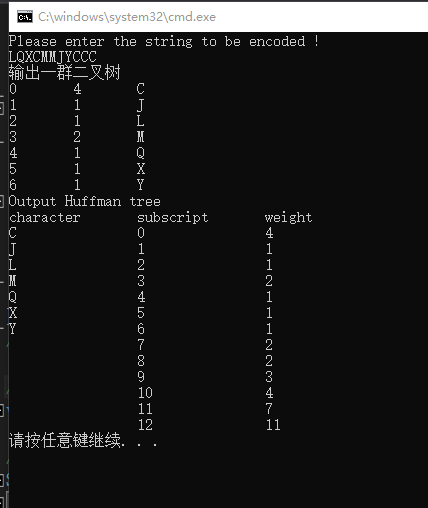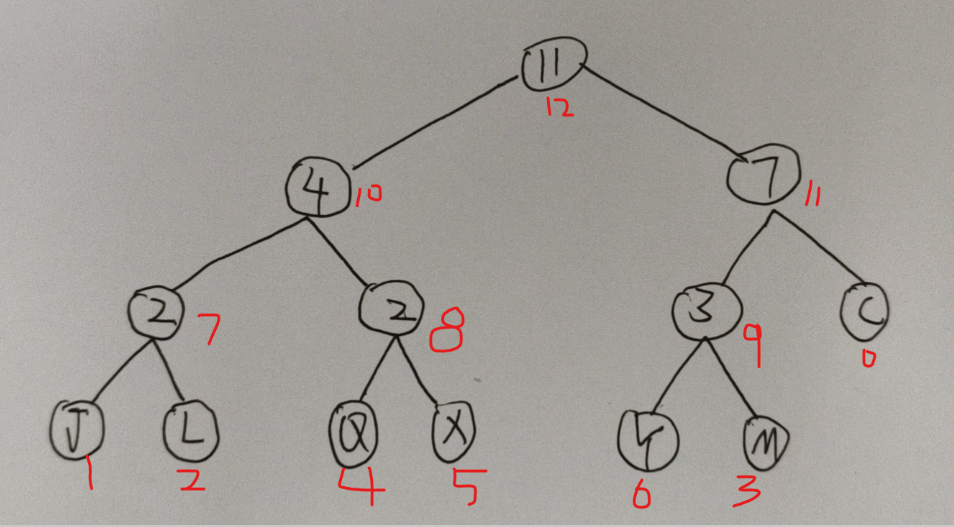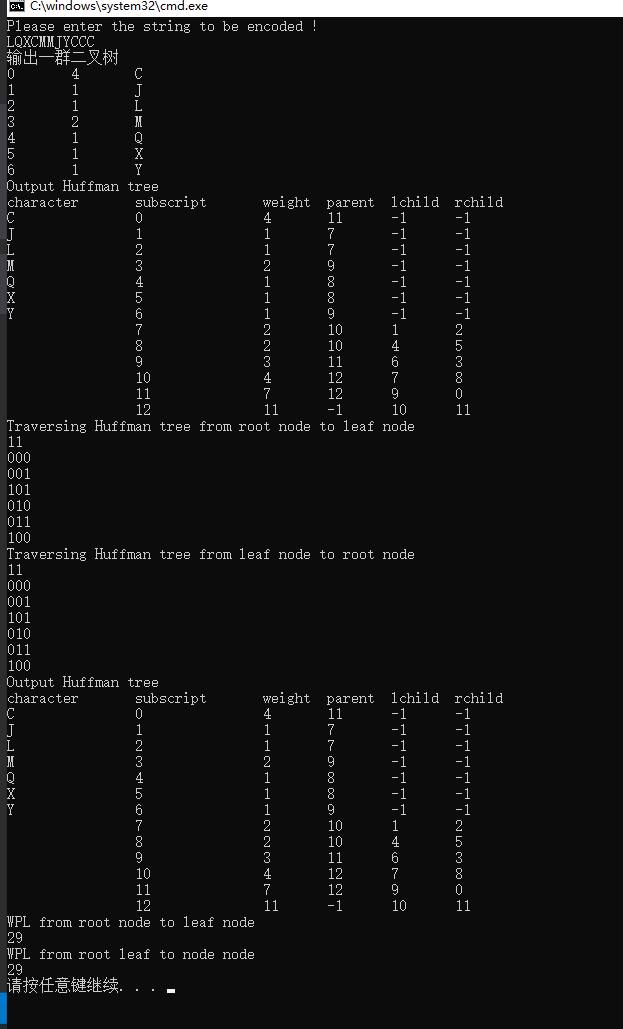• 在visual studio平台，利用c语言结合优先队列等数据结构，实现哈夫曼树编码与解码
• 哈夫曼树T1 T2 T37 aca b c d 4 d 2 5 b7 5 2 4 7 a b 5 2 c d 4问...

哈夫曼树
T1 T2 T3
7 a
c
a b c d 4 d 2 5 b
7 5 2 4 7 a b 5 2 c d 4
问题
什么是哈夫曼树？
树结构 解放军理工大学
编码
编码：是指用不同的0 、1序列代表不同的信息。
 西文信息(ASCII码)
 汉字信息 (GB2312编码、GBK 、BIG5)
 图像、图形、声音等信息
树结构 解放军理工大学
编码方案
总的原则
 要能唯一地译码
 编码长度要尽量短
树结构 解放军理工大学
编码种类
等长编码
不等长编码
树型编码 哈夫曼编码
树结构 解放军理工大学
哈夫曼
戴维·哈夫曼(David A. Huffman 1925—1999)
美国计算机科学家，分别于1944年和1949年从俄亥俄
洲立大学获得学士和硕士学位，1953年在麻省理工学院
(MIT )获得博士学位。1962—1967年在MIT任教授，1967
年到加洲大学圣克鲁斯分校创办计算机系。他将他全部的精
力放在教学上，以他自己的话来说，“我所要带来的就是我
的学生。”
1982年获得IEEE计算机先驱奖，所提出的哈夫曼编码方
法被广泛应用于数据的压缩和传输。
树结构 解放军理工大学
哈夫曼树
教学目标和要求
1. 能够准确阐述哈夫曼树、哈夫曼编码的定义
2. 能够描述哈夫曼树的自底向上子树合并构造算法思想
3. 对于给定的编码频度，能够正确给出其哈夫曼编码
4.能够举例说明哈夫曼编码应用
5.能够编程实现哈夫曼树构造算法
树结构 解放军理工大学
哈夫曼树相关术语
叶的路径长度 从根结点到叶结点的路径上的边数。
A 的路径长度： 2
D
B 、C 的路径长度：3
A
D 的路径长度： 1
B C
树结构 解放军理工大学
哈夫曼树相关术语
叶的加权路径长度 设二叉树的叶子，其权值为正实数
w ，路径长度为l ，那么，W*l 称为

展开全文• VC++2012编程演练数据结构《24》哈夫曼树
• 这篇博客只是简单介绍一下具体题目哈夫曼树的应用，其实就是堆的部分应用，在这题里面维护的是一个最小堆。详细的堆排可以看这里。 1. 定义： 给定n个权值作为n个叶子结点，构造一棵二叉树，若该树的带权路径长度...
这篇博客只是简单介绍一下具体题目哈夫曼树的应用，其实就是堆的部分应用，在这题里面维护的是一个最小堆，写的并不仔细。细节可以点击这里堆以及堆的堆的操作 1. 定义： 给定n个权值作为n个叶子结点，构造一棵二叉树，若该树的带权路径长度达到最小，称这样的二叉树为最优二叉树，也称为哈夫曼树(Huffman Tree)。哈夫曼树是带权路径长度最短的树，权值较大的结点离根较近。 2. 作用： 哈夫曼编码 来看一下具体题目吧 Problem Description 字符的编码方式有多种，除了大家熟悉的ASCII编码，哈夫曼编码(Huffman Coding)也是一种编码方式，它是可变字长编码。该方法完全依据字符出现概率来构造出平均长度最短的编码，称之为最优编码。哈夫曼编码常被用于数据文件压缩中，其压缩率通常在20%～90%之间。你的任务是对从键盘输入的一个字符串求出它的ASCII编码长度和哈夫曼编码长度的比值。 Input 输入数据有多组，每组数据一行，表示要编码的字符串。 Output 对应字符的ASCII编码长度la，huffman编码长度lh和la/lh的值(保留一位小数)，数据之间以空格间隔。 Sample Input AAAAABCD THE_CAT_IN_THE_HAT Sample Output 64 13 4.9 144 51 2.8 直接上码了
#include<stdio.h>
#include<string.h>
int size,a,b;
void in(int x)
{
int i,t;
a[++size]=x;
for(i=size; i!=0; i/=2)
{
if(a[i]<a[i/2])
{
t=a[i];
a[i]=a[i/2];
a[i/2]=t;
}
else
break;
}
}
void delete()
{
int p,i,t;
a = a[size--];
for(i = 1; i*2 <= size; i = p)
{
if(a[i*2+1] < a[i*2] && i*2+1 <= size)
p = i*2+1;
else
p = i*2;
if(a[p] < a[i])
{
t = a[i];
a[i] = a[p];
a[p] = t;
}
else
break;
}
}
int main()
{
char s;
int n,sum,la,ans,i;		//ans即lh
double fna;
while(~scanf("%s",s))
{
ans=0;		//多组输入记得初始化就行
size=0;
memset(b,0,sizeof(b));
memset(a,0,sizeof(a));
n=strlen(s);
la=n*8.0;
for(i=0; i<n; i++)
{
b[s[i]]++;
}
for(i=0; i<=500; i++)
{
if(b[i]!=0)
in(b[i]);
}
while(size>1)
{
int x=a;
delete();
int y=a;
delete();
sum=x+y;
in(sum);
ans+=sum;
}
fna=(double)(la)/(double)(ans);
printf("%d %d %.1lf\n",la,ans,fna);
}
return 0;
}

展开全文• 输入任意字符串，统计不同字符个数，进行哈夫曼编码


哈夫曼编码实现
需实现的功能输入不同字符串并统计生成哈夫曼树给哈夫曼树进行编码
完整编程

需实现的功能
//实现的功能
//1.输入一行字符串 找出各个字符出现的次数 及其对应的字符，将其保存在二叉树中，组成森林//或者组成二叉树
//2.从森林中挑选权值最小的两颗的合并，且这两颗作为新树的左右树，（左小右大），且新树的权值为此两颗树之和。
//3.从森林中删除那两颗树，并将新树加入
//4.重复2 3两步 直至森林中树为1。
//5.加编码
//6.返回权值
//7.解码


这里实现了 看代码咯
输入不同字符串并统计
功能：输入任何字符  统计每个不同字符的个数


cout << "Please enter the string to be encoded !" << endl;
fflush(stdin);

//string s;   //字符串 区别在于不能够输入空格的ASCII码
//cin>> s;

char s[MAX];//能输入空格  不过要提前定义一个足够大数组
cin.get(s,MAX);

//概率初始化
//int *probabiblity = new int;
for (int i = 0; i < 128; i++)
{
probabiblity[i] = 0;
}

/*for (int i = 0; i < s.size(); i++)//检验字符串
{
cout << s[i] << endl;
}*/

//统计0-128个字符中出现的次数  按照ASCII码排序
for (int j = 0; s[j] != '\0'; j++)
{
//cout << s[j] << endl;
probabiblity[s[j]]++;//这里我的理解是pro[] 里面是int型    放过字符 强制转换成对应的int类型
}

//统计不同字符数   sum计数
for (int k = 0; k < 128; k++)
{
if (probabiblity[k] != 0)
{
sum++;//不同字符数目
//cout <<k<<'\t'<< probabiblity[k] <<(char)k<< endl;
}
else
continue;
}
return 0;
}

生成哈夫曼树
一开始输入字符也在生成哈夫曼树里的
但是为了更方便 将其拆开了

首先将保存字符数量信息的数组改成从零开始
！！！！这里我的操作是按ASCII码排序  而不是按出现的次数排序


Huffman CreatTree(Huffman *Tree,int *probabiblity,int &sum)
{
/*
int sum = 0;
string s;
cout << "Please enter the string to be encoded !" << endl;
fflush(stdin);
cin >> s;

//概率初始化
int *probabiblity = new int;
for (int i = 0; i < 128; i++)
{
probabiblity[i] = 0;
}

/*for (int i = 0; i < s.size(); i++)//检验字符串
{
cout << s[i] << endl;
}*/
/*
//统计0-128个字符中出现的次数  按照ASCII码排序
for (int j = 0; s[j] !='\0'; j++)
{
//cout << s[j] << endl;
probabiblity[s[j]]++;
}

//统计不同字符数   sum计数
for (int k = 0; k < 128; k++)
{
if (probabiblity[k] != 0)
{
sum++;//不同字符数目
//cout <<k<<'\t'<< probabiblity[k] <<(char)k<< endl;
}
else
continue;
}
//cout << sum << endl;
*/
//sum=Enterstring(probabiblity);

/*
int sum=0;
int *probabiblity = new int;
Enterstring(probabiblity,sum);*/
//HuffmanCode Tree;//但是这样好像比较占内存  后续改改
//Huffman *Tree = (Huffman*)malloc((2*sum-1)* sizeof(HTNODE));
int t,x=0;

//用数组保存树的结构信息 将数组序号改成从0依次增加。
for (int k = 0; k < 128; k++)
{
if (probabiblity[k] != 0)					//k是0-128之间对应着ASCII码。
{
t = k;									//k的值用t保存
Tree[x] = new HuffmanTree;				//将x取代数组序号k
Tree[x]->lchild = -1; //就是因为一开始将孩子信息定义为整数型 导致后面没应用递归
Tree[x]->rchild = -1;  //如果孩子结点是树的结构体指针  那么用递归会方便很多
Tree[x]->parent = -1;
Tree[x]->symbol = (char)t;	//也是将int型强制转换为char型			//元素  合成的结点 就没有放元素
Tree[x]->weigth = probabiblity[t];		//权重  即次数
x++;

//上面的树 如果不加数组的话  每次循环都会有变化，应该每次都将这些树加入到一个序列当中！！！或者组成森林   但是还是没有排序
//先尝试编一下森林的
}
else
continue;
}
free(probabiblity);//释放空间
//输出二叉树看看
cout << "输出一群二叉树" << endl;  //没问题
for (int k = 0; k < sum; k++)
{

cout << k << '\t' << Tree[k]->weigth <<'\t'<< Tree[k]->symbol << endl;

}
//完成了单个二叉树的构造

int min1, min2;								//min 为左树数组序号    max为右树数组序号
//sum  为需要编码的叶子数目
for (int j = sum; j < 2 * sum - 1; j++)
{
SortTree(Tree,j, min1, min2);//这里需要找到一个能够将数组中找出权值最小的两颗树，并删除此两颗树,然后生成一个权值为二者之和的新树。整体-2+1。当树的数目为1是停止。
Tree[min1]->parent = j;
Tree[min2]->parent = j;

Tree[j] = new HuffmanTree;//动态
Tree[j]->symbol = NULL;
/*Tree[j]->lchild = Tree[min1];
Tree[j]->rchild = Tree[min2];*/
Tree[j]->lchild = min1;
Tree[j]->rchild = min2;
Tree[j]->weigth = Tree[min1]->weigth + Tree[min2]->weigth;
Tree[j]->parent = -1;//这一步开始忘记了  导致不能使得新结点进入排序
}
//需要编码的多个信息都已经保存到了对应不同的二叉树中
cout << "Output Huffman tree" << endl;  //没问题
cout << "character" << '\t' << "subscript"  <<'\t' << "weight" << endl;
for (int k = 0; k < 2*sum-1; k++)
{
cout<<Tree[k]->symbol << '\t'<<'\t' << k <<'\t'<< '\t' << Tree[k]->weigth << endl;
}
return *Tree;
}给哈夫曼树进行编码
这里搜索的还不太熟悉 然后自己想又一时想不出了 纠结了很久唉 提醒一下自己！！！ 后面加上编码的时候一时没有加上，后来看了其他的代码 还是这方面练习的少啊
如上图所示，每个结点都有数组下标，而且需要编码的字符都在最前面
所以可以先从第一个点开始找
比如先找到C结点，其数组下标为0
用current记录此时下标为0；
然后我们可以找到C的双亲结点（权值为7）用father记录其数组下标（11）

再判断current是father的左孩子还是右孩子
左孩子记录为0；右孩子记录为1

如何记录呢 用新的数组，（需要编码的长度最长不超过   不同字符数-1）
又因为是从尾部开始的 所以code[--start]

循环寻找每一个不同的字符位置

code是一个中间保存编码的值
start每次都不同  且编码在后面
所以用strcmp(H_code,code+start)复制过去

//从根结点到叶子结点遍历哈弗曼树 生成哈夫曼编码
Status HuffmanCode1(Huffman *Tree, Huffmancode &H_Code,int &sum)
{
H_Code = (Huffmancode)malloc(sum * sizeof(char *));//记录编码
char *cd = (char*)malloc(sum * sizeof(char));//
//int temp, count;
cd[sum - 1] = '\0';//哈夫曼编码最长为需要编码不同字符-1；
int i;
for (i = 0; i < sum; i++)
{
int current = i;
int father = Tree[i]->parent;
int start = sum - 1;
while (father != -1)
{
if (Tree[father]->lchild == current)
{
cd[--start] = '0';
}
else
{
cd[--start] = '1';
}
current = father;
father = Tree[father]->parent;
}
H_Code[i] = (char *)malloc((sum - start) * sizeof(char));
strcpy(H_Code[i], cd + start);
}
for (int i = 0; i < sum; ++i) {
cout << H_Code[i] << endl;;
}
free(cd);
return 0;
}


在用strcmp的时候遇到错误解决办法——在最前面加上这个
#pragma warning( disable : 4996)

//从根结点到叶子结点遍历哈弗曼树 生成哈夫曼编码

Status HuffmanCode2(Huffman *Tree,Huffmancode &H_Code,int &sum)
{
cout <<"Traversing Huffman tree from leaf node to root node" << endl;
H_Code = (Huffmancode)malloc(sum * sizeof(char *));							//保持编码信息
char *cd = (char*)malloc(sum * sizeof(char));								//生成编码的过渡信息
int *a = (int *)malloc((2 * sum - 2 + 1) * sizeof(int));					//保存权值信息

int cur = 2 * sum - 2;
int cd_len = 0;

for (int i = 0; i < cur + 1; i++)											//以权值作为信息
{																			//权值信息都为0   然后用数组a保存原权值
a[i] = Tree[i]->weigth;
Tree[i]->weigth = 0;

}

//这里要好好看看
//编码过程
while (cur != -1)
{

if (Tree[cur]->weigth == 0)//权值为0时说明没有进行遍历
{
Tree[cur]->weigth = 1;//权值为1 说明进行了左遍历
if (Tree[cur]->lchild != -1)//NULL：空指针， 在C++中值为0，在C中定义为(void *)0.
{
cd[cd_len++] = '0';
cur = Tree[cur]->lchild;
}
else
{
cd[cd_len] = '\0';
H_Code[cur]= (char *)malloc((cd_len + 1) * sizeof(char));
strcpy(H_Code[cur],cd);
}
}
else if (Tree[cur]->weigth == 1)
{
Tree[cur]->weigth = 2;//权值为2进行了右遍历
if (Tree[cur]->rchild != -1)
{
cd[cd_len++] = '1';
cur = Tree[cur]->rchild;
}
}
else
{
//Tree[cur]->weigth = 0;
cur = Tree[cur]->parent;//结束标志
--cd_len;
}
}

for (int i = 0; i < 2 * sum - 2 + 1; i++)
{
Tree[i]->weigth= a[i];//还原权值
}

for (int i = 0; i < sum; ++i) {//输出编码
cout << H_Code[i] << endl;;
}
free(cd);
free(a);
return 0;
}

完整编程
#pragma warning( disable : 4996)
#include<iostream>
#include<string>
#include <cstring>
#include<malloc.h>
using namespace std;
#define MAX 100
typedef int Status;
typedef char element;
//实现的功能
//1.输入一行字符串 找出各个字符出现的次数 及其对应的字符，将其保存在二叉树中，组成森林//或者组成二叉树
//2.从森林中挑选权值最小的两颗的合并，且这两颗作为新树的左右树，（左小右大），且新树的权值为此两颗树之和。
//3.从森林中删除那两颗树，并将新树加入
//4.重复2 3两步 直至森林中树为1。
//5.加编码
//6.返回权值
//7.解码
typedef struct HuffmanTree
{
int weigth;
int parent;
char symbol;
//struct HuffmanTree *lchild, *rchild;
int lchild, rchild;
}HTNODE,*Huffman;

//typedef char **HCode;
typedef char **Huffmancode;

Status Enterstring(int *probabiblity, int &sum);
Huffman CreatTree(Huffman *Tree, int *probabiblity, int &sum);
void SortTree(Huffman *Tree, int j, int &min1, int &min2);
Status find_D_min(Huffman *tree, int j);
Status HuffmanCode1(Huffman *Tree, Huffmancode &H_Code, int &sum);
Status HuffmanCode2(Huffman Tree, Huffmancode &H_Code, int &sum);
Status WPL1(Huffman *Tree, int &sum);
Status WPL1(Huffman *Tree, int &sum);

//因为需要知道需要编码数 n  所以需要把字符串输入这一个函数单独写出来
Status Enterstring(int *probabiblity,int &sum)
{
cout << "Please enter the string to be encoded !" << endl;
fflush(stdin);

//string s;   //字符串 区别在于不能够输入空格的ASCII码
//cin>> s;

char s[MAX];//能输入空格  不过要提前定义一个足够大数组
cin.get(s,MAX);

//概率初始化
//int *probabiblity = new int;
for (int i = 0; i < 128; i++)
{
probabiblity[i] = 0;
}

/*for (int i = 0; i < s.size(); i++)//检验字符串
{
cout << s[i] << endl;
}*/

//统计0-128个字符中出现的次数  按照ASCII码排序
for (int j = 0; s[j] != '\0'; j++)
{
//cout << s[j] << endl;
probabiblity[s[j]]++;
}

//统计不同字符数   sum计数
for (int k = 0; k < 128; k++)
{
if (probabiblity[k] != 0)
{
sum++;//不同字符数目
//cout <<k<<'\t'<< probabiblity[k] <<(char)k<< endl;
}
else
continue;
}
return 0;
}

Huffman CreatTree(Huffman *Tree,int *probabiblity,int &sum)
{
/*
int sum = 0;
string s;
cout << "Please enter the string to be encoded !" << endl;
fflush(stdin);
cin >> s;

//概率初始化
int *probabiblity = new int;
for (int i = 0; i < 128; i++)
{
probabiblity[i] = 0;
}

/*for (int i = 0; i < s.size(); i++)//检验字符串
{
cout << s[i] << endl;
}*/
/*
//统计0-128个字符中出现的次数  按照ASCII码排序
for (int j = 0; s[j] !='\0'; j++)
{
//cout << s[j] << endl;
probabiblity[s[j]]++;
}

//统计不同字符数   sum计数
for (int k = 0; k < 128; k++)
{
if (probabiblity[k] != 0)
{
sum++;//不同字符数目
//cout <<k<<'\t'<< probabiblity[k] <<(char)k<< endl;
}
else
continue;
}
//cout << sum << endl;
*/
//sum=Enterstring(probabiblity);

/*
int sum=0;
int *probabiblity = new int;
Enterstring(probabiblity,sum);*/
//HuffmanCode Tree;//但是这样好像比较占内存  后续改改
//Huffman *Tree = (Huffman*)malloc((2*sum-1)* sizeof(HTNODE));
int t,x=0;

//用数组保存树的结构信息 将数组序号改成从0依次增加。
for (int k = 0; k < 128; k++)
{
if (probabiblity[k] != 0)					//k是0-128之间对应着ASCII码。
{
t = k;									//k的值用t保存
Tree[x] = new HuffmanTree;				//将x取代数组序号k
Tree[x]->lchild = -1;
Tree[x]->rchild = -1;
Tree[x]->parent = -1;
Tree[x]->symbol = (char)t;				//元素
Tree[x]->weigth = probabiblity[t];		//权重  即次数
x++;

//上面的树 如果不加数组的话  每次循环都会有变化，应该每次都将这些树加入到一个序列当中！！！或者组成森林   但是还是没有排序
//先尝试编一下森林的
}
else
continue;
}
free(probabiblity);//释放空间
//输出二叉树看看
cout << "输出一群二叉树" << endl;  //没问题
for (int k = 0; k < sum; k++)
{

cout << k << '\t' << Tree[k]->weigth <<'\t'<< Tree[k]->symbol << endl;

}
//完成了单个二叉树的构造

int min1, min2;								//min 为左树数组序号    max为右树数组序号
//sum  为需要编码的叶子数目
for (int j = sum; j < 2 * sum - 1; j++)
{
SortTree(Tree,j, min1, min2);//这里需要找到一个能够将数组中找出权值最小的两颗树，并删除此两颗树,然后生成一个权值为二者之和的新树。整体-2+1。当树的数目为1是停止。
Tree[min1]->parent = j;
Tree[min2]->parent = j;

Tree[j] = new HuffmanTree;
Tree[j]->symbol = NULL;
/*Tree[j]->lchild = Tree[min1];
Tree[j]->rchild = Tree[min2];*/
Tree[j]->lchild = min1;
Tree[j]->rchild = min2;
Tree[j]->weigth = Tree[min1]->weigth + Tree[min2]->weigth;
Tree[j]->parent = -1;
}
//需要编码的多个信息都已经保存到了对应不同的二叉树中
cout << "Output Huffman tree" << endl;  //没问题
cout << "character" << '\t' << "subscript"  <<'\t' << "weight" <<'\t'<<"parent"<< '\t' <<"lchild"<< '\t' << "rchild"<< endl;
for (int k = 0; k < 2*sum-1; k++)
{
cout<<Tree[k]->symbol << '\t'<<'\t' << k <<'\t'<< '\t' << Tree[k]->weigth << '\t' <<Tree[k]->parent<< '\t' <<Tree[k]->lchild<<'\t'<<Tree[k]->rchild<< endl;
}
return *Tree;
}
//这里需要找到一个能够将数组中找出权值最小的两颗树，并删除此两颗树  ，然后生成一个权值为二者之和的新树   整体-2+1    当树的数目为1是停止。

//找到树组前j个权值最小的两个
void SortTree(Huffman *Tree,int j,int &min1,int &min2)
{
min1 = find_D_min(Tree, j);
min2 = find_D_min(Tree, j);
//return 0;
}

//找到前k个树中最小的一个 然后返回该数组序号
Status find_D_min(Huffman *tree, int j)
{
int min, min_wei, i = 0;

while (tree[i]->parent != -1)			//找到第一个合法值 好和后面做比较
{
i++;
}
min_wei = tree[i]->weigth;				//相当于把一堆树中第一个合法的权值赋予它  然后排序找最小zhi
min = i;								//此时数组序号

for (; i < j; i++)
{
if (tree[i]->weigth < min_wei&&tree[i]->parent == -1)
{
min_wei = tree[i]->weigth;
min = i;
}

}

tree[min]->parent = 0;					//排除最小的一个

return min;
}

//从叶子点到根结点遍历二叉树 生成哈夫曼编码
Status HuffmanCode1(Huffman *Tree, Huffmancode &H_Code,int &sum)
{
cout << "Traversing Huffman tree from root node to leaf node" << endl;
H_Code = (Huffmancode)malloc(sum * sizeof(char *));
char *cd = (char*)malloc(sum * sizeof(char));

cd[sum - 1] = '\0';//哈夫曼编码最长为需要编码不同字符-1；

int i;
for (i = 0; i < sum; i++)
{
int current = i;
int father = Tree[i]->parent;
int start = sum - 1;
while (father != -1)
{
if (Tree[father]->lchild == current)
{
cd[--start] = '0';
}
else
{
cd[--start] = '1';
}
current = father;
father = Tree[father]->parent;
}
H_Code[i] = (char *)malloc((sum - start) * sizeof(char));
strcpy(H_Code[i], cd + start);
}
for (int i = 0; i < sum; ++i) {
cout << H_Code[i] << endl;;
}
free(cd);
return 0;
}

//从根结点到叶子结点遍历哈弗曼树 生成哈夫曼编码
Status HuffmanCode2(Huffman *Tree,Huffmancode &H_Code,int &sum)
{
cout <<"Traversing Huffman tree from leaf node to root node" << endl;
H_Code = (Huffmancode)malloc(sum * sizeof(char *));							//保持编码信息
char *cd = (char*)malloc(sum * sizeof(char));								//生成编码的过渡信息
int *a = (int *)malloc((2 * sum - 2 + 1) * sizeof(int));					//保存权值信息

int cur = 2 * sum - 2;
int cd_len = 0;

for (int i = 0; i < cur + 1; i++)											//以权值作为信息
{																			//权值信息都为0   然后用数组a保存原权值
a[i] = Tree[i]->weigth;
Tree[i]->weigth = 0;

}

//这里要好好看看
//编码过程
while (cur != -1)
{

if (Tree[cur]->weigth == 0)//权值为0时说明没有进行遍历
{
Tree[cur]->weigth = 1;//权值为1 说明进行了左遍历
if (Tree[cur]->lchild != -1)//NULL：空指针， 在C++中值为0，在C中定义为(void *)0.
{
cd[cd_len++] = '0';
cur = Tree[cur]->lchild;
}
else
{
cd[cd_len] = '\0';
H_Code[cur]= (char *)malloc((cd_len + 1) * sizeof(char));
strcpy(H_Code[cur],cd);
}
}
else if (Tree[cur]->weigth == 1)
{
Tree[cur]->weigth = 2;//权值为2进行了右遍历
if (Tree[cur]->rchild != -1)
{
cd[cd_len++] = '1';
cur = Tree[cur]->rchild;
}
}
else
{
//Tree[cur]->weigth = 0;
cur = Tree[cur]->parent;//结束标志
--cd_len;
}
}

for (int i = 0; i < 2 * sum - 2 + 1; i++)
{
Tree[i]->weigth= a[i];//还原权值
}

for (int i = 0; i < sum; ++i) {//输出编码
cout << H_Code[i] << endl;;
}
free(cd);
free(a);
return 0;
}

//从叶子结点返回wpl
Status WPL1(Huffman *Tree, int &sum)
{
int i, countRoads, WPL = 0;//如果需要知道每个叶子的带权路径长度  定义一个数组分别保存即可
//数组前sum都是信息
for (i = 0; i < sum; i++)
{
//每次都从结点从新开始计算wpl
int father = Tree[i]->parent;
while (father != -1)
{
father = Tree[father]->parent;//找到根结点就跳出     与编码过程不同的是  编码时需要有确认孩子是左孩子还是右孩子
}
}
return WPL;
}

//从根结点到叶子
Status WPL2(Huffman *Tree, int &sum)
{
int cur = 2 * sum - 2;//数组最大值

int *visit = (int *)malloc((2*sum-2)*sizeof(int));

for (int i = 0; i < cur+1; i++)
{
visit[i] = 0;
}

while (cur!= -1)//结束时返回到根部
{
if (visit[cur] == 0)
{
visit[cur] = 1;//遍历左路径 设置为1
if (Tree[cur]->lchild != -1)
{
//左孩子存在时
cur = Tree[cur]->lchild;//路径加1   cur换孩子坐标
}
else
{
}
}
else if (visit[cur] == 1)
{
//已经遍历了左孩子  然后开始遍历右孩子
visit[cur] = 2;
if (Tree[cur]->rchild != -1)
{
cur = Tree[cur]->rchild;
}
}
else
{
//左右孩子均遍历
visit[cur] = 0;  //这个改不改都无所谓
cur = Tree[cur]->parent;
}
}
return WPL;
}
int main()
{
//输入字符串(所有英文格式的字符串)
int sum = 0;
int *probabiblity = new int;
Enterstring(probabiblity, sum);
//创造哈夫曼树
Huffman *Tree = (Huffman*)malloc((2 * sum - 1) * sizeof(HTNODE));
CreatTree(Tree,probabiblity,sum);
//从根节点到叶子结点遍历二叉树 生成哈夫曼编码
Huffmancode H_Code;
HuffmanCode1(Tree, H_Code, sum);
HuffmanCode2(Tree, H_Code, sum);
//输出
cout << "Output Huffman tree" << endl;  //没问题
cout << "character" << '\t' << "subscript" << '\t' << "weight" << '\t' << "parent" << '\t' << "lchild" << '\t' << "rchild" << endl;
for (int k = 0; k < 2 * sum - 1; k++)
{
cout << Tree[k]->symbol << '\t' << '\t' << k << '\t' << '\t' << Tree[k]->weigth << '\t' << Tree[k]->parent << '\t' << Tree[k]->lchild << '\t' << Tree[k]->rchild << endl;
}
cout << "WPL from root node to leaf node" << endl;
cout << WPL1(Tree, sum) << endl;
cout << "WPL from root leaf to node node" << endl;
cout << WPL2(Tree, sum) << endl;
return 0;
}

结果：感觉哈夫曼还用了不少时间 一是能力还不够 二是拔牙然后周末摸鱼 白天学代码不自在 哎呀 希望寒假效率高一点 （可能吗@！！！？？冲）
这次借鉴了一下大佬的代码
哈夫曼树及哈夫曼编码详解【完整版代码】
然后还有一些没有涉及到 （哈夫曼解码 用栈的结构 ） 后面再学学不同方法 哈夫曼报文编码和译码（涨姿势！！！） 哈夫曼树(C语言简单实现） 哈夫曼编码的C语言实现
展开全文数据结构 算法 指针
• 网上有不少人介绍哈夫曼树的生成方法，但是对于实现方式却都只字不提，只是放了代码，这里简单介绍一下一个我自己编写的哈夫曼树的实现方式。 如果你完全不知道哈夫曼树的生成方式，那么本篇文章并不适合你阅读，...
网上有不少人介绍哈夫曼树的生成方法，但是对于实现方式却都只字不提，只是放了代码，这里简单介绍一下一个我自己编写的哈夫曼树的实现方式。 如果你完全不知道哈夫曼树的生成方式，那么本篇文章并不适合你阅读，如果你已经了解哈夫曼树的构造方式，但是并不知道如何使用C/C++实现，那么请继续阅读。 哈夫曼树生成时，首先需要将2个weight最小的树叶组队，再新建一个parents给两个树叶，然后一直循环，直到构建完成。 那么，其实可以将结构体数组当成leaves和节点，构建树的实际操作就是构建每个数组元素的联系，如何将数组元素联系起来？ 用结构体数组，每个数组元素都有lchild和rchild，以及一个sw，child就不说了，用来存与自己联系的两个数组元素下表，那么sw是用来干嘛的呢？ 用来“删除”该元素。因为在构建树的时候，已经拥有了parents的元素不再参与weight比较以及构建，而是他们的parents参与，所以需要有一个属性来将他们“关闭” parents和leaves的类型相同，拥有的属性也相同，并且parents不能复用，每个参与构建树的parents并非真的被“删除”了而是被“关闭”了，这个概念很重要。 需要编码N个字符，需要的数组大小为2N-1个，但是最开始开启的数组元素只有N个，当parents元素找到自己的两个child之后才会开启，并参与下次的weight比较。 解码等我我有空说，自己想想应该也能想出来了。
展开全文c++
• 本文主要是对哈夫曼树代码进行介绍，感性趣的朋友可以参考下。
• 2 掌握建立哈夫曼树和哈夫曼编码的方法及带权路径长度的计算。  【设计内容】   欲发一封内容为AABBCAB „„(共长 100 字符,其中:A 、B 、C 、D 、E 、F分别有7 、9 、12 、22 、23 、27个)的电报...数据结构课设
• ## 哈夫曼树、哈夫曼编码详解

千次阅读 多人点赞 2021-06-17 09:33:06
哈夫曼树、哈夫曼编码，也就这么一回事，一文搞懂！算法 哈夫曼编码 数据结构
• 哈夫曼树 哈夫曼树又称最优二叉树, 是一种带权路径长度最短的二叉树。在这个二叉树中，只有叶子节点才是有效的数据节点,其他只是作为路径而构造的。 带权路径长度 又称WPL，树的带权路径长度，就是树中所有的叶结点...算法 哈夫曼编码
• 哈夫曼树和哈夫曼编码 1.哈夫曼树的定义： 在许多实际应用之中，树中节点常常被赋予一个表示某种意义的数值，称为该结点的权。从树根结点到任意结点的路径长度（经过的边数）与该结点上权值的乘积，称为该结点的带权...哈夫曼编码
• #include<stdio.h> #define M 8 #define MAX 200 ...void huffman(int n,int w[],JD t[]) //用一维结构数组存储，由N个节点构成的哈夫曼树，节点数为2N-1，叶子节点数为N { int i,j,k,x1,x2,m1
• 8609 哈夫曼树 时间限制:1000MS 代码长度限制:10KB 提交次数:3178 通过次数:1263 题型: 编程题 语言: G++;GCC Description 利用静态链表建立赫夫曼树，建树过程中要求左子树权值小于右子树权值，求各结点的编码。...二叉树
• ## 【笔记】哈夫曼树

千次阅读 2017-11-01 00:34:54
哈夫曼树的概念 哈夫曼树的构造算法 哈夫曼编码 哈夫曼编码算法的实现哈夫曼编码 哈夫曼算法 最优二叉树
• 大学数据结构课程设计，使用哈夫曼树进行压缩和解压缩，C语言编程
• Java哈夫曼编码实验–哈夫曼树的建立，编码与解码 建树，造树，编码，解码 一、哈夫曼树编码介绍 1、哈夫曼树： （1）定义：假设有n个权值{w1, w2, …, wn}，试构造一棵含有n个叶子结点的二叉树，每个叶子节点...
• ## 哈夫曼树的创建

千次阅读 2017-06-09 15:37:25
什么是哈夫曼树？ 路径和最短的二叉树 如何构造最优二叉树，也就是哈夫曼树 哈夫曼树的构建过程？ 1.给出一个数组，以每个元素为基础，创建一个叶子节点数组。 2.从中找出权值最小的两个节点，构建一棵...二叉树
• 很明显 这是一道构造一棵哈夫曼树。具体的构造如下所示。参考代码。 #include #include #include #include #include using namespace std; struct huffman{ int sum; int leftc; int rightc; ...
• 建立一棵二叉链表树，分别输出此先根、中根和后根遍历序列 将上题编程，实现哈夫曼树的构建和哈夫曼编码的设计
•二叉树 算法 数据结构
• 哈夫曼树 满二叉树 完全二叉树 平衡二叉树 表达式树 红黑树 B+ B- AVL ... 1.二叉树的组成 根节点 子节点（左字节点，右子节点，双亲节点） 叶子节点（终端节点） 树的...
•数据结构 java...